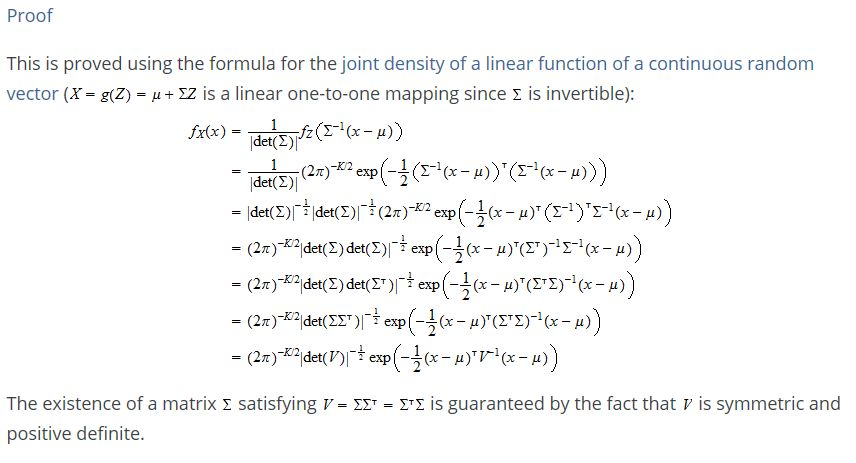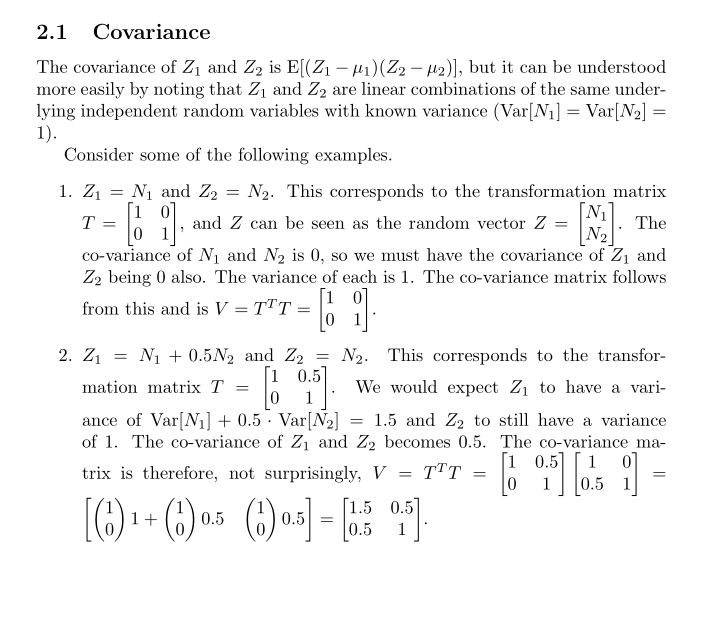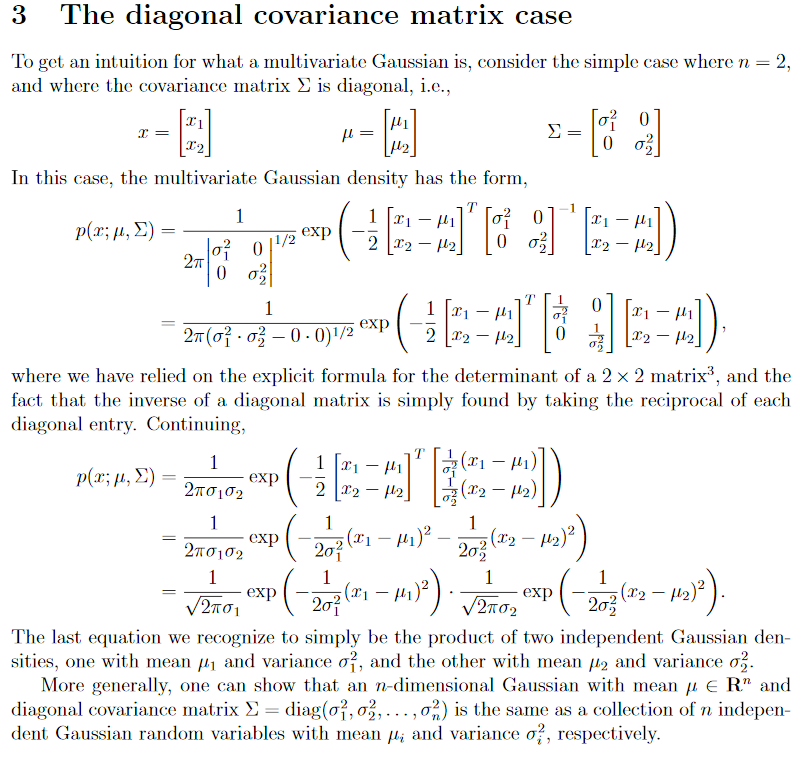Show Question
Math and science::INF ML AI

# Multivariate Gaussian distribution

The general multivariate normal distribution can be conceptualized as the distribution of a random variable which is a linear transformation of a another random variable having a standard multivariate normal distribution.

### Standard multivariate Gaussian/normal distribution

Let $$(\Omega, \mathrm{F}, \mathbb{P})$$ be a probability space. Let $$X : \Omega \to \mathbb{R}^K$$ be a continuous random vector. $$X$$ is said to have a standard multivariate normal distribution iff its joint probability density function is:

$f_X(x) = (\frac{1}{\sqrt{2\pi} })^K e^{-\frac{ x^\mathsf{T}x }{2} }$

#### As a vector of random variables

$$X$$ can be considered to be a vector of independent random variables, each having a standard normal distribution. The proof of this formulation on the reverse side.

### General multivariate

The general multivariate normal distribution is best understood as being the distribution that results from applying a linear transformation to a random variable having a multivariate standard normal distribution.

### General multivariate normal distribution

Let $$(\Omega, \mathrm{F}, \mathbb{P})$$ be a probability space, and let $$Z : \Omega \to \mathrm{R}^K$$ be a random vector with a multivariate standard normal distribution. The let $$X = \mu + \Sigma Z$$ be another random vector. $$X$$ has a distribution $$f_X : \mathbb{R}^K \to \mathbb{R}$$ which is a transformed version of $$Z$$'s distribution, $$f_Z : \mathbb{R}^K \to \mathbb{R}$$:

\begin{alignat*}{3}f_X(x) &= \frac{1}{\text{rescaling factor} } \; &&f_z(\text{z in terms of x}) \\&= \frac{1}{|\operatorname{det}(\Sigma )|} &&f_z(\Sigma^{-1}(x - \mu)) \\ &= \frac{1}{|\operatorname{det}(\Sigma )|} &&(\frac{1}{\sqrt{2\pi} })^K e^{\textstyle \frac{(\Sigma^{-1}(x - \mu))^\mathsf{T}(\Sigma^{-1}(x - \mu))}{2} } \\ \end{alignat*}

#### Standard multivariate normal as a vector of independent random variables. Proof.

Proof that the above probability density function represents $$K$$ independent standard normal random variables. For clarity, only the case when $$K = 3$$ will be highlighted:
\begin{aligned} f_Z(z) &= \frac{1}{ (2\pi)^{ \frac{3}{2} } } e^{-\frac{z^Tz}{2}} \\ &= \frac{1}{ (2\pi)^{\frac{1}{2}} } \frac{1}{(2\pi)^{\frac{1}{2}}} \frac{1}{(2\pi)^{\frac{1}{2}}} e^{-\frac{z^Tz}{2}} \\ &= \frac{1}{(2\pi)^{\frac{1}{2}}} \frac{1}{(2\pi)^{\frac{1}{2}}} \frac{1}{(2\pi)^{\frac{1}{2}}} e^{-\frac{z_1^2 + z_2^2 + z_3^3}{2}} \\ &= \frac{1}{\sqrt{2\pi}} \frac{1}{\sqrt{2\pi}} \frac{1}{\sqrt{2\pi}} e^{-\frac{z_1^2}{2}} e^{-\frac{z_2^2}{2}} e^{-\frac{z_3^2}{2}} \\ &= \frac{1}{\sqrt{2\pi}} e^{-\frac{z_1^2}{2}} \frac{1}{\sqrt{2\pi}} e^{-\frac{z_2^2}{2}} \frac{1}{\sqrt{2\pi}} e^{-\frac{z_3^2}{2}} \\&= f(z_1) f(z_2) f(z_3) \end{aligned}

Thus, the propability distribution for $$Z$$ represents 3 independent random variables having a individual distribution of $$\frac{1}{\sqrt{2\pi}}e^{-\frac{z_i^2}{2}}$$ which is the standard normal distribution for a single random variable.

### Elementwise conceptualization

The quantity $$[X - \mu] \Sigma^{-1} [X-\mu]$$ can be thought of as a component wise operation, sum($$\frac{[X - \mu ]^2}{\sigma^2}$$), where $$\sigma$$ is the column vector of standard deviations. Another alternative is to imagine the component wise methods that would be called in a linear algebra/DL library: accumulate(mult(invert(pow($$\sigma$$, 2)), pow($$X - \mu$$), 2))).

#### $$V = \Sigma^{\mathsf{T}} \Sigma$$ form. Proof.

The Statlect formulation simplifies the expression. Copy-pasted here:### Dispelling the mystery of the co-variance matrix

Here is a screenshot of some notes on the perspective of a multivariate Gaussian random variable being a composition of two standard normal variables. It tries to dispell some of the mystery about why the co-variance matrix comes up the way it does.### Example